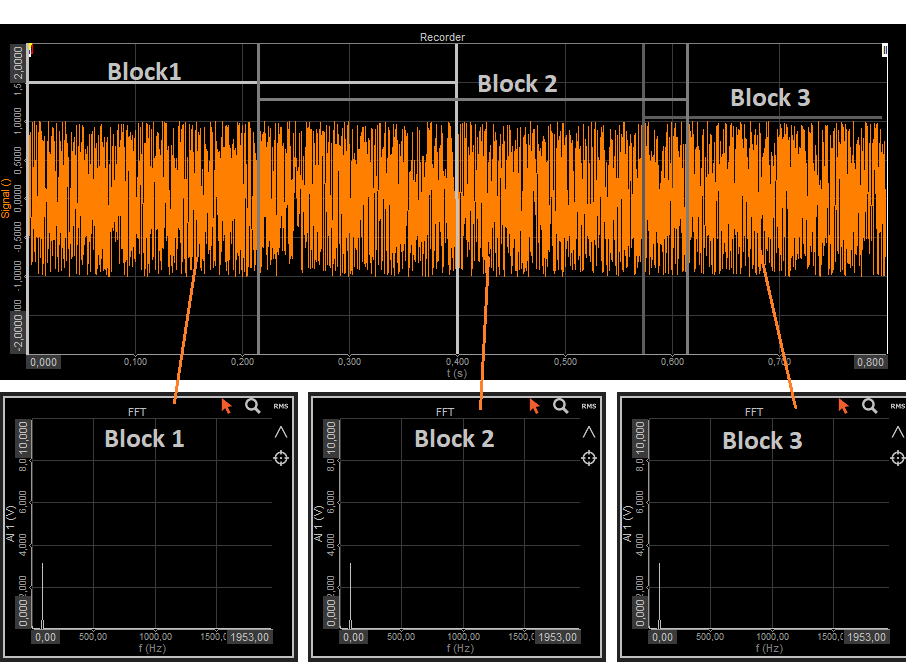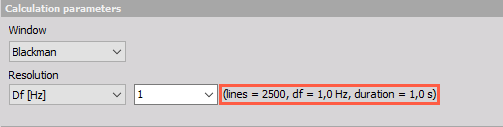FFT visual control

IN MEASUREMENT

Line resolution:

Adjusted by user from 256 to 64k number of lines.

The block duration:

The duration of the calculation block depends on the display update rate which can be adjusted in settings. The visual control will take 1/50 seconds of time domain data and peform FFT analysis.This is only the preferred display update rate and can vary depending on CPU and GPU load and on the setup complexity.

Number of samples in each block is calculated from line resolution:

number of samples = line resolution x 2 = 1024 lines x 2 =2048 samples

Overlap can be calculated out of display update rate and block duration.

Example:

If we use the sample rate 10kHz, line resolution 1024 lines and display update rate 50Hz, this is how we can calculate the overlap.

Block duration = 1/50Hz = 0,02s

Block duration in samples = 0,02s x 10000 = 200 samples

Block samples = 2 x 1024 = 2048

Overlap = 1 - (Block duration in samples/Block samples) = 1-(200/2048) = 90,23%

Because of all the variables in this equation, the overlap is changing during measurement.

The block sizes are of the same length throughout the measurement, but they are not equidistant.

Sample rate: 50kHz

Resolution: 256 lines

Block duration: 0,01s

Display refresh rate: 50Hz(0,02s) <-this is not a fixed valueSample rate: 50kHz

Resolution: 1024 lines

Block duration: 0,04s

Display refresh rate: 50Hz(0,02s) <-this is not a fixed valueIN ANALYSIS:

The only thing that can be adjusted in analysis is line resolution from 256 to 64k. The size of the block will be always 2 x line resolution and Dewesoft will show results in relation to the position of the yellow cursor. Because the FFT data is not stored during measurement Dewesoft will recalculate the data when the cursor is moved.FFT analyser

IN MEASUREMENT:

Line resolution:

User can adjust number of lines (128 to 64k), define block duration or frequency resolution.

The block duration:

The block duration is calculated from the acquisition sample rate and number of lines. In the channel setup Dewesoft will also show the calculated values:Number of samples in each block is calculated from line resolution:

block samples = line resolution x 2 -> 2500 lines x 2 =5000 samples

Overlap can be adjusted separately and doesn't affect the calculated block.

IN ANALYSIS:

Because all the calculated data is stored during the measurement, the data is completely the same in the analysis. If any parameters are changed in the mathematics, offline recalculation is needed.

Comparison of the FFT visual control and FFT analyser is hard, because the parameters are never completely the same.